# Linear Regression - find the perfect line: Pearssons Correlation Coefficient, Covariance, Variance, Standard Deviation¶

In :
from sklearn.datasets import load_boston
import pandas as pd

#get data

#convert data to DataFrame
data = pd.DataFrame(boston_dataset.data, columns=boston_dataset.feature_names)

data['MEDV'] = boston_dataset.target

#print first 5 rows of data

Out:
CRIM ZN INDUS CHAS NOX RM AGE DIS RAD TAX PTRATIO B LSTAT MEDV
0 0.00632 18.0 2.31 0.0 0.538 6.575 65.2 4.0900 1.0 296.0 15.3 396.90 4.98 24.0
1 0.02731 0.0 7.07 0.0 0.469 6.421 78.9 4.9671 2.0 242.0 17.8 396.90 9.14 21.6
2 0.02729 0.0 7.07 0.0 0.469 7.185 61.1 4.9671 2.0 242.0 17.8 392.83 4.03 34.7
3 0.03237 0.0 2.18 0.0 0.458 6.998 45.8 6.0622 3.0 222.0 18.7 394.63 2.94 33.4
4 0.06905 0.0 2.18 0.0 0.458 7.147 54.2 6.0622 3.0 222.0 18.7 396.90 5.33 36.2
In :
#single feature RM as x-variable
x_variable = data['RM']

#target variable
y_variable = data['MEDV']


### Analytical solution¶

In the main tutorial we learned about the idea of how to find the best fitting line for our data: A line with the least amount of squared errors. But how exactly is this line calculated? For a better understanding, let's start with the very basics - the equation for a line. $$y = mx + b$$ The "m" defines the slope of the line and "b" the intercept. Ok, now we know the mathematical definition of a line. Now we have to think of the line that fits our data best. In order to do that let's first forget about this line and think about the equation of squared errors. $$MSE=\frac{1}{n}\sum\limits_{j=1}^n(y_i - \hat{y_i})^2$$ It is a square function so from its derivative we can calculate the slope at every point of the function. We want the lowest point of the squared error function because that is where the sum of the squared errors is the least. The lowest point of the equation of squared errors is where the slope is zero. Thus, the derivative is set to zero. Well, actually we have two variables which we want to find out: the slope and the intercept. Therefore, we first calculate the partial derivative of the intercept (b). Then set it to zero. Then solve the equation for "b". We then replace "b" in the partial derivative of the slope "m" which is also set to zero. We then use a lot of fancy mathmatical tricks and in the end we have the two equations to calculate "b" and "m":

$$b = mean(y) - m(mean(x))$$$$m = \frac{covariance(X,Y)}{variance(X)}$$

The equation of "m" can also be expressed as the following:

$$m = \frac{r\:(standarddeviation(Y)}{standarddeviation(X)}$$

"r" is the Pearson Correlation Coefficient - a really cool indicator of how strongly correlated two variables are. We talked about it in the main tutorial when we wanted to find out which variable has strong effects on our target variable. In order to learn how to calculate "r" we use the second equation in order to find "m". Nevertheless, it usually makes more sense to use the first one since it causes slightly less computational costs.

First, we have a look at how the covariance of X and Y can be calculated. The result indicates if X and Y are linearly dependent. We received a positive result, so there is a positive correlation between X and Y.

In :
import numpy as np

#mean of x values
x_mean = np.mean(x_variable)

#mean of y values
y_mean = np.mean(y_variable)

#caluculates the covariance of X and Y
#param: x_variable: x-vlaues
#param: y_variable: y-values
#return: cov: covariance
def covariance_caluclation(x_variable, y_variable):
cov = 0
x_mean = np.mean(x_variable)
y_mean = np.mean(y_variable)

for i in range (0,len(x_variable)):
cov += (x_variable[i]-x_mean)*(y_variable[i]-y_mean)

cov = cov/len(x_variable)

return cov

cov = covariance_caluclation(x_variable, y_variable)

print(cov)

4.484565551719291


We then calculate the variance of both, X and Y. The variance is the squared distance of each data point to the mean of the data points divided by the total number of data points. By extracting the square root of the variance we get the standard deviation.

In :
import math

#calculates the variance of a feature/the target variable
#param: values: X or Y values
#return: variance: variance of a feature/the target variable depending on the parameter input
def calculate_variance(values):
mean_values = np.mean(values)
sum_squared_distance_to_mean = 0

for i in range (0, len(values)):
sum_squared_distance_to_mean += (values[i]-mean_values)**2

variance = sum_squared_distance_to_mean/len(values)
return variance

#variance of X
x_variance = calculate_variance(x_variable)

#standard deviation of X
x_standard_deviation = math.sqrt(x_variance)

#variance of Y
y_variance = calculate_variance(y_variable)

#standard deviation of Y
y_standard_deviation = math.sqrt(y_variance)

print(x_standard_deviation)
print(y_standard_deviation)

0.7019225143345692
9.188011545278206


The previous calculations help us to calculate Pearson's Correlation Coefficient "r". The result is around 0.7 which shows a positive correlation between our x and y-variables.

In :
#Pearson's correlation coefficient
r = cov/(x_standard_deviation*y_standard_deviation)
print(r)

0.6953599470715389


Using our functions we can now calculate the y-intercept "b" and the slope "m" and finally build our equation.

In :
#calculation slope
m = r*(y_standard_deviation/x_standard_deviation)
print(m)

#calculation intercept
b = y_mean - m*x_mean
print(b)

#x-values
x = x_variable

#equation
y = m*x+b

9.102108981180303
-34.67062077643857


Of course, we would also like to see the result! So let's plot our line together with the x-variables. Wow, look at this! We just calculated the best fitting line totally by ourselves! Fantastic!

In :
import matplotlib.pyplot as plt

#definiton of figure size
fig=plt.figure(figsize=(8, 8))

#plot data points
plt.scatter(x_variable, y_variable,  marker='o', s=6, color = "black")

#name y-axis
plt.ylabel('Median value of owner-occupied homes in \$1000')

#name x-axis
plt.xlabel('Number of rooms')

#plot line
plt.plot(x,y)

Out:
[<matplotlib.lines.Line2D at 0x1a1a75f7f0>]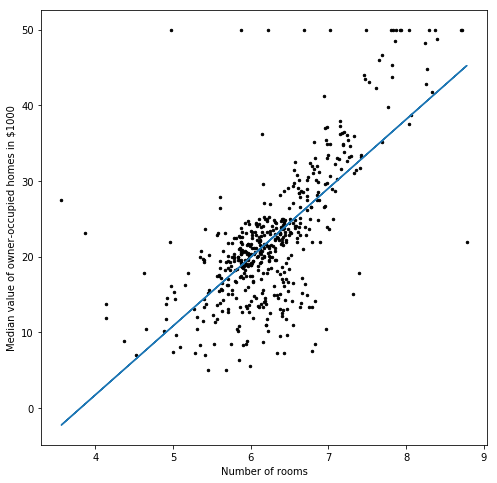Great! Now we know about the analytical approach to find the best line! Let's also learn about the numerical approach. What's the differnece between an analytical and a numerical approach? With the analytical approach it is possible to find an exact result. Nevertheless, sometimes it is not possible to find the exact solution or it can take a really long time and a lot of computational costs to find the exact solution. In our example we only had one feature and one dimension so an analytical approach was still possible but often this is not the case. Therefore, with the numerical approach we try to find an approximation to the solution. This can also take some time but it is usually much faster and of course better than not finding any solution at all.

We will learn about Gradient Descent - a numerical optimization algorithm that tries to find the local minimum of a function. Gradient Descent is used in most of machine learning algortihms and we will learn how it works by looking at a simple Linear Regression model with one feature and the target variable.

Therefore, we firstly create a few data points and plot them. We can already see that a simple Linear Regression would be a good model to fit our data.

In :
import matplotlib.pyplot as plt

#creat some x-values
x_values = [-10,-9,-5,-2,-1, 2,3,6,8, 9,11]

#creat some x-values
y_values = [-9,-6,-4, -3,-2, 3,5,8,10,10,13]

#plot the data
plt.scatter(x_values,y_values)
plt.show()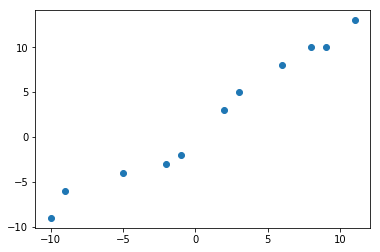This next function is actually not really necessary in order to calculate the best values for slope and the y-intercept for our line. It is just for us in order to find out if the Gradient Descent algorithm which we are about to implement actually does the job it is supposed to do or not.

As we already learned - the whole idea about how to find the perfect line is about reducing the mean squared error. Thus, we just use this function to be able to store all the mean sqaured errors of all the lines on our way to find the line that fits our data best. If everything goes well then the mean squared error of the first line, which was just an initial guess, should far exceed the mean squared error of our last line. Unless, of course, we are just amazingly good at guessing.

In :
def calculate_mean_squared_error(intercept, slope, x_values, y_values):
mean_squared_error = 0
for i in range (0,len(y_values)):
mean_squared_error += (y_values[i] - (intercept+slope*x_values[i]))**2
mean_squared_error = mean_squared_error/len(y_values)
return mean_squared_error


Now we use the partial derivatives of the mean squared error function. As we have already learned, we want to find values for the slope and the y-intercept that reduce the mean squared error as far as possible. The lowest point would be where the combination of the partial dervatives of the slope (m) and the intercept (b) of the error function euqals zero. A Gradient is just the combination of several partial derivatives of a function. Since we want to find the minimum of our loss function by using this combination of partial derviatives, the algortihm is called Gradient Descent. Now that we solved this secret let's apply the Chain Rule to find the partial derivatives of the slope and the intercept. If you have not already solved this - no worries - here is the partial derivative with respect to the intercept : $$\frac{\partial}{\partial b} = \sum\limits_{j=1}^n \frac{-2}{n}(y_i-\hat{y_i})$$

This can also be expressed this way: $$\frac{\partial}{\partial b} = \sum\limits_{j=1}^n\:\frac{-2}{n}(y_i-(b+mx_i)$$

The following function returns the value of the slope of the error function that has been calculated by plugging in some random guess for the slope and some random guess for the intercept into the partial derivative with respect to the intercept.

In :
def calculate_intercept_derivative(intercept,slope, x_values, y_values):
for i in range (0,len(y_values)):


Let's now calculate the partial derivative with respect to the slope (m). Are you ready? Great! Then here is the result: $$\frac{\partial}{\partial m}=\sum\limits_{j=1}^n\frac{(-2)}{n}\:x_i\:(y_i-\hat{y_i})$$

That is just the same as: $$\frac{\partial}{\partial m} = \sum\limits_{j=1}^n\:\frac{(-2)}{n}\:x_i\:(y_i-(b+mx_i)$$

In :
def calculate_slope_derivative(intercept,slope, x_values, y_values):
for i in range (0,len(y_values)):


The next function is just as unnecessary for the Gradient Descent algorithm as the mean squared error function. It is just for us, so that we know all the y-values of all the lines on our way while trying to find the best line. We will just use it for some visualization later.

In :
def calculate_line_derivative(y_derivative_slope, y_derivative_intercept, x_values, y_values):
y_derivative=[]
for i in range (0,len(y_values)):
y_derivative.append(y_derivative_slope*x_values[i] + y_derivative_intercept)
return y_derivative


This function is basically what the Gradient Descent is all about and what makes it different from the analytical solution: The values we get from the partial derivatives by plugging in our values for the slope and the intercept are now being used in order to calculate a new slope and a new intercept that work better than the previous ones. How is this being done? In order to understand how it works let's first define a few terms:

Step Size: A number which defines how far we move into the right direction while trying to find the best intercept and the best slope for our line. Let's say we found a slope using the partial derivative of the intercept. If we had found the perfect values for m and b this would be zero. Usually it is not and we then multiply it by something called a Learning Rate. The result of this multiplication is then the Step Size.

Minimum Step Size: The minimum Stepsize to continue the Gradient Descent algortihm

Learning Rate: Usually a very small value that is being multiplied with the result of the partial derivatives after having plugged in our values.

Maximum amount of Trials: Maximum amount of iterations for the Gradient Descent algortihm

Not clear so far? No worries let's look at an example:

Let's take the procedure for the intercept as an example - the exact same thing is then being done with the slope:

Let's say we define as a first try the following variables:
m = 1
b = 0
learning_rate = 0.01

Then we plug the values into the partial derivative with respect to the intercept. We also plug in our values for x and y. $$\frac{\partial}{\partial b} = \sum\limits_{j=1}^n\:\frac{(-2)}{n}(y_i-(0+1x_i)$$ Let's say the number we get as a result is 7. Then we multiply this number with our Learning Rate. $$stepsize = 7\cdot0.01 = 0.07$$ As a result we get our Step Size. We subtract the Step Size from our previous intercept and get the new intercept: $$b_{new} = b-stepsize = 0-0.07 = -0.07$$ The same procedure is done in order to find the new and better slope (m).

In :
def calculate_new_line_variable(variable, y_derivative, learning_rate):
new_variable = variable-y_derivative*learning_rate
return new_variable


Well, now we just put all the previously explained functions together and put them in a loop which only stops when either the Minimum Step Size or the Maximum amount of Trials is reached.

In :
def gradient_descent(intercept, slope, learning_rate, x_values, y_values, min_step_size, max_trials):
line_information = []
count = 0

intercept_gradient = calculate_intercept_derivative(intercept, slope, x_values, y_values)
slope_gradient = calculate_slope_derivative(intercept, slope, x_values, y_values)

mean_squared_error = calculate_mean_squared_error (intercept, slope, x_values, y_values)
line_information.append({'mean_squared_error':mean_squared_error, 'intercept':intercept, 'slope':slope, 'derivative_y_values':derivative_y_values})

intercept = new_intercept
slope = new_slope

count+=1
return line_information, slope, intercept, count


Now let's test if the line with the values for the slope and the intercept (blue) looks better than the line with the initial guesses we made (red).

Hurray! It really looks perfect! Also the number of Mean Squared Errors shrinked tremendously! Fantastic!

In :
learning_rate = 0.01
min_step_size = 0.0000000000001
max_trials = 200
intercept_first_try = -5
slope_first_try = -3

line_information, slope, intercept, count = gradient_descent(intercept_first_try, slope_first_try, learning_rate, x_values, y_values, min_step_size, max_trials)

print('Number of iterations ' + str(count))
print('Mean Squared Error with initial guesses ' + str(line_information['mean_squared_error']))
print('Mean Squared Error after Gradient Descent '+ str(line_information[-1]['mean_squared_error']))

print('intercept ' + str(intercept))
print('slope ' + str(slope))

y_first_line = calculate_line_derivative(slope_first_try, intercept_first_try, x_values, y_values)

y_new_line = calculate_line_derivative(slope, intercept, x_values, y_values)

plt.scatter(x_values, y_values)
plt.plot(x_values, y_new_line)
plt.plot(x_values, y_first_line, color = "red")

Number of iterations 200
Mean Squared Error with initial guesses 868.7272727272727
Mean Squared Error after Gradient Descent 1.4017997282527697
intercept 1.034293332043501
slope 1.0296919657524752

Out:
[<matplotlib.lines.Line2D at 0x1a235f24a8>]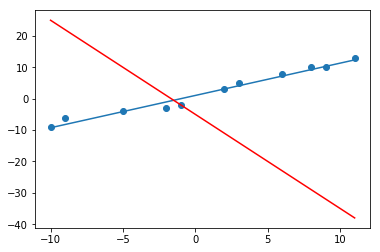Let's also have a look at how quickly the lines improved by displaying some of them while finding the best fitting line. From being totally wrong to already being very close to the best line is being done within one step here. One great advantage of Gradient Descent is that the steps are very big in the beginning until they become very little when getting closer to the best possible line. This - at least most of the times - prevents the algorithm from overstepping the best possible result.

In :
plt.scatter(x_values, y_values)

for i in range (0,80, 15):
intercept = line_information[i]['intercept']
slope = line_information[i]['slope']
line = calculate_line_derivative(slope, intercept, x_values, y_values)
plt.plot(x_values, line)

plt.plot(x_values, y_new_line)

plt.show()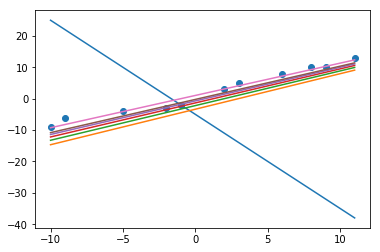Of course, we would also like to have a 3D visualization of the Gradient Descent. Therefore, let's get a few more values for the Mean Squared Error for various values for the slope and the intercept. Since our algorithm stores all those information in a list we just have to run it again. Since we started with negative values for the intercept and the slope last time, let's start with postivie values now in order to get a nice 3D plot.

In :
line_information_2, slope_2, intercept_2, count_2 = gradient_descent(9, 5, learning_rate, x_values, y_values, min_step_size, max_trials)
line_information_1 = line_information
line_information.extend(line_information_2)

intercept_values = []
slope_values = []
mean_squared_errors = []
for item in line_information:
intercept_values.append(item['intercept'])
slope_values.append(item['slope'])
mean_squared_errors.append(item['mean_squared_error'])


In the 3D plot we can see why taking the partial derivatives was that important: Only the combination of both, the pefect value for the slope and the perfect value for the intercept gives us the acutal minimum of our loss function.

In :
from mpl_toolkits.mplot3d import Axes3D

z = mean_squared_errors

fig1 = plt.figure(figsize=(10,10))

ax1 = Axes3D(fig1)

ax1.set_xlabel('slope')
ax1.set_ylabel('intercept')
ax1.set_zlabel('mean squared error')

surf = ax1.plot_trisurf(slope_values, intercept_values, z,
cmap='magma')

plt.show()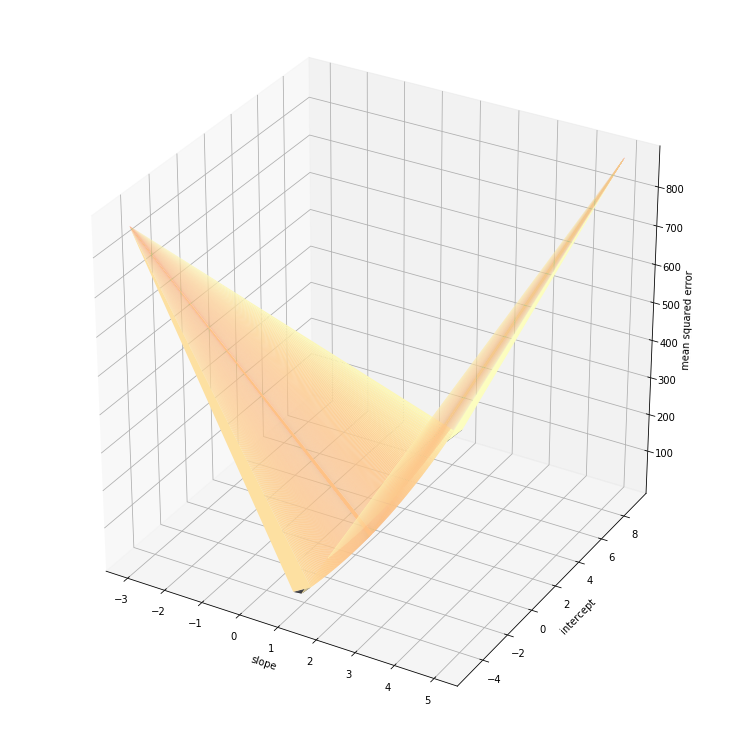I hope you liked this tutorial - see you next time!